### Though powerful enough to run a business, spreadsheets are simple enough for everyone to quickly and easily learn to use. Here we take you through the things you have to know about spreadsheets and functional thinking.

Numbersense: Multiplying & Dividing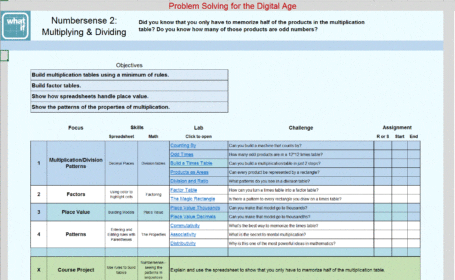Did you know that you only have to memorize half of the products in the multiplication table? Do you know how many of those products are odd numbers, and why that is important?

Numbersense: Primes, Integers, Powers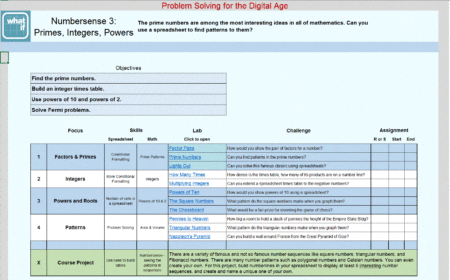The prime numbers are among the most interesting ideas in all of mathematics. Can you use a spreadsheet to find patterns to them?

Ratio 1: Decimals, %, Fractions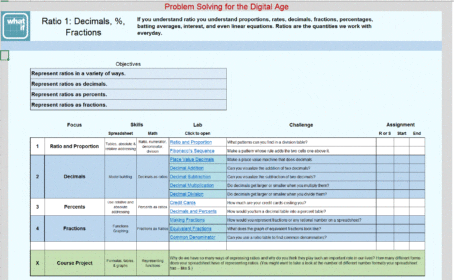If you understand ratio you understand proportions, rates, decimals, fractions, percentages, batting averages, interest, and even linear equations. Ratios are the quantities we work with everyday.

Ratio 2: Interest, Rate, Finance, Functions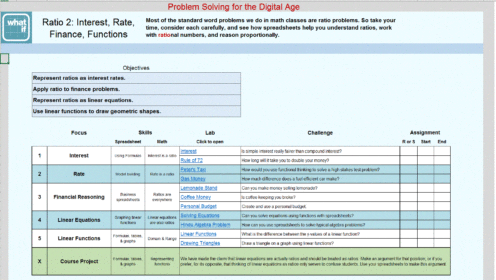Most of the standard word problems we do in math classes are ratio problems. So take your time, consider each carefully, and see how spreadsheets help you understand ratios, work with rational numbers, and reason proportionally.

Classic Story Problems

These are the problems typically found in traditional arithmetic and algebra courses and tests. Spreadsheets give us a new way to solve them, a way that shows their common form, makes them concrete, and enables you to find the right answer even when you do not have access to a computer.

Financial Reasoning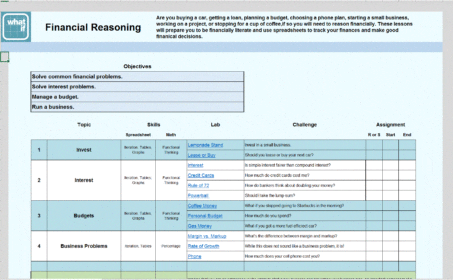Whether you are buying a car, getting a loan, planning a budget, choosing a phone plan, starting a small business, working on a project, or stopping for a cup of coffee, you will need to reason financially. These Labs prepare you to be financially literate, to use spreadsheets to track your finances, and make good financial decisions.

Functions 1: Linear & Exponential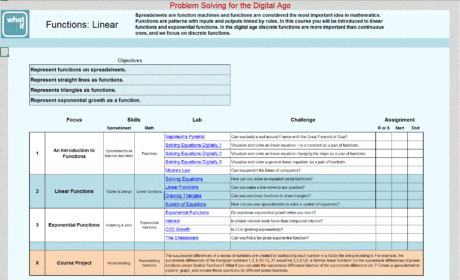Spreadsheets are function machines and functions are considered the most important idea in mathematics. Functions are patterns with inputs and outputs linked by rules. In this course you will be introduced to linear functions and exponential functions. In the digital age discrete functions are more important than continuous ones, and spreadsheets are a great platform for learning to use discrete functions.

Functions 2: Families & Operations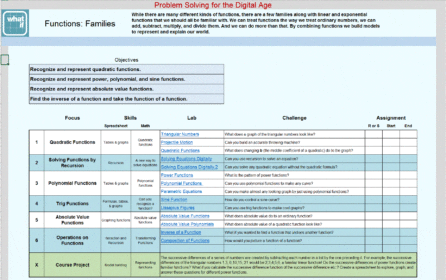Though there are many different kinds of functions, there are a few kinds along with linear and exponential functions that you should be familiar with. One of the most powerful aspects of functions is that we can treat them with the same operations we use with ordinary numbers: we can add, subtract, multiply, and divide them, and even take a function of a function. Combining functions using these operations enable us to build wonderful models to represent and explain our world.

Patterns & Coding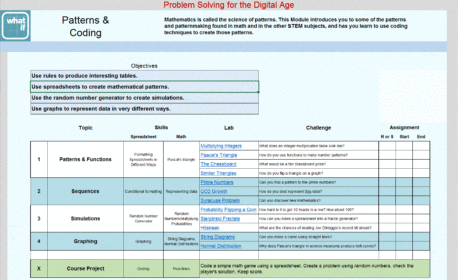Mathematics, Though powerful enough to run a big business is called the science of patterns. This module introduces you to some of the patterns and patternmaking found in math and in the other STEM subjects.ess, spreadsheets are simple enough for everyone to quickly and easily learn to use. Here we take you through the things you have to know about spreadsheets and functional thinking.